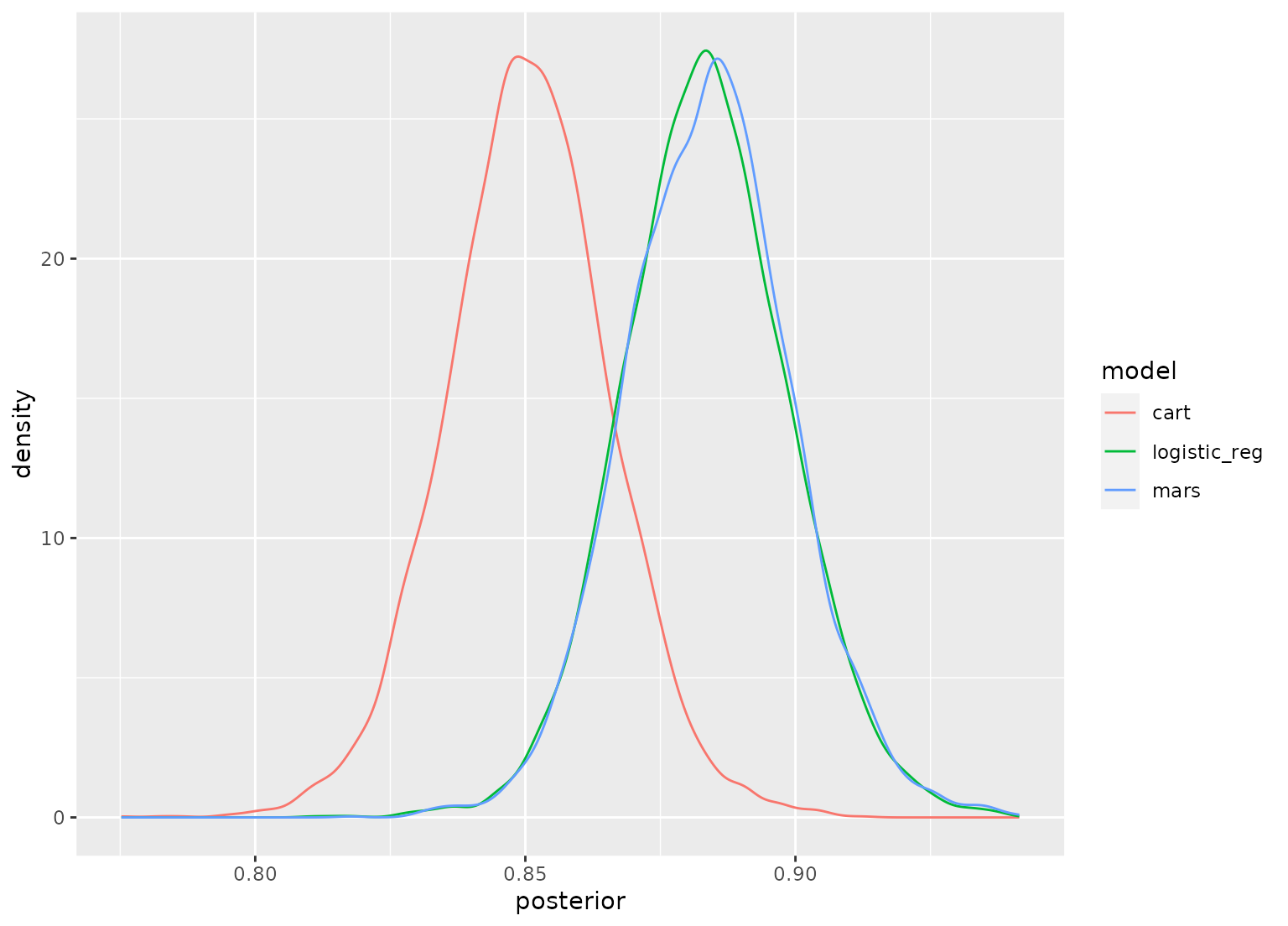For objects of classes posterior and perf_mod, autoplot() produces a simple plot of posterior distributions. For workflow set objects, there are several types of plots that can be produced.

# S3 method for posterior
autoplot(object, ...)

# S3 method for perf_mod
autoplot(object, ...)

# S3 method for perf_mod_workflow_set
autoplot(object, type = "intervals", prob = 0.9, size = NULL, ...)

## Arguments

object An object produced by perf_mod(), tidy.perf_mod(), or a workflow set with computed results. Options passed to geom_line(stat = "density", ...). A value of one of: "intervals" (for model rank versus posterior probability using interval estimation), "posteriors" (density plots for each model), or "ROPE" (for practical equivalence probabilities versus workflow rank). A number p (0 < p < 1) indicating the desired probability mass to include in the intervals. The size of an effective difference in the units of the chosen metric. For example, a 5 percent increase in accuracy (size = 0.05) between two models might be considered a "real" difference.

## Value

A ggplot2::ggplot() object.

## Examples

data(ex_objects)
autoplot(posterior_samples)